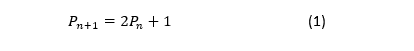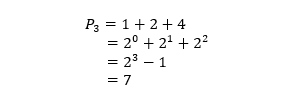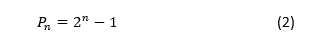By Maria Kapsis, University of South Australia

The birth of the Tower of Hanoi puzzle occurred in 1883 when French mathematician, Edouard Lucas, published his series of four volumes Récréations mathématiques. The name ‘Tower of Hanoi’ refers to the capital city of Vietnam. When Lucas started to market the puzzle, French newspapers were full of reports about the siege that France had on the city of Hanoi against the Chinese. Hinz [1, p.6] makes the conclusion that Lucas selected the name of Hanoi because it was in the headlines at the time.

The puzzle comprises a pile of discs of decreasing radius sitting on one of three pegs. The aim is to transfer the pile of discs to another peg, moving only one disc at a time, without letting a bigger disc rest on a smaller one. I was first introduced to this puzzle in high school by a very inspiring maths teacher who taught me that simple mathematical concepts can provide the best explanations to puzzling problems. The puzzle has a recursive solution, given by Hinz [1, p.10], which can be applied for an arbitrary number of discs. If the puzzle can be solved for eight discs, then it can be solved for nine discs by transferring the upper eight to another peg, then moving the ninth disc to the final peg and finally transferring the remaining eight discs to the final peg. If the number of moves required to transfer n discs is P_n then to move n+1 discs you need to move n discs, then move the largest discs, then move n discs again. This can be written asThe first three solutions are P_0=0 (it takes no moves to move no discs), P_1=1, then P_2=3.

There is another way to count the number of moves required, by focusing on the number of times each disc is moved. Take for example the solution for 3 discs. We know that the largest disc only is required to move once, the second largest disc moves twice and the smallest moves four times. This equates to a total of seven moves.The number of moves corresponding to each disc, reveals a binary number sequence, 2^0+2^1+2^2+⋯.

This is because for every disc that is added, the smallest disc doubles the amount of moves each time. The number of moves iswhich is the solution to the recursive equation in (1).

 A.M. Hinz, S. Klavzar, U. Milutinovic, and C. Petr. The Tower of Hanoi – Myths and Maths. Springer Basel, Heidelberg, New York, Dordrecht, London, 2013.

This book writes about how the game originated and includes the formulated solutions mentioned in this blog.

Maria Kapsis was a recipient of a 2018/19 AMSI Vacation Research Scholarship.